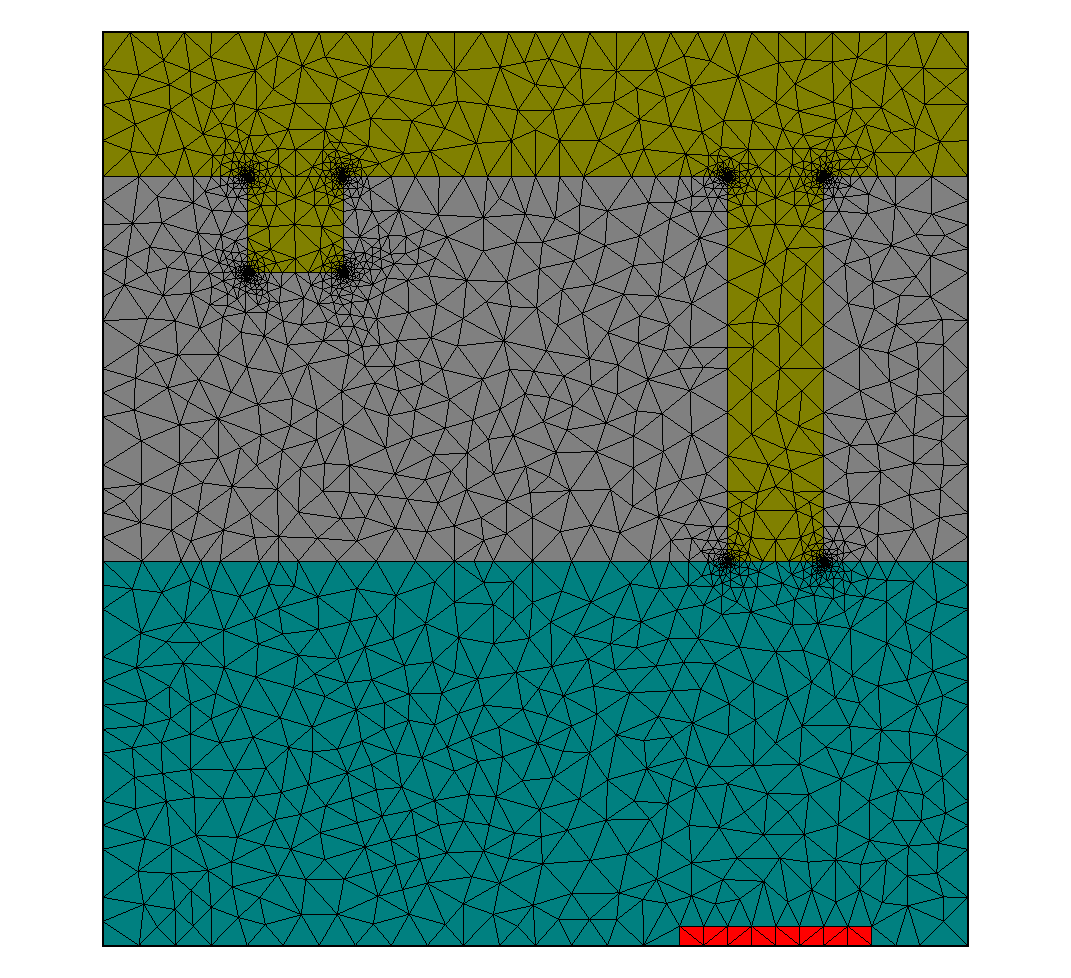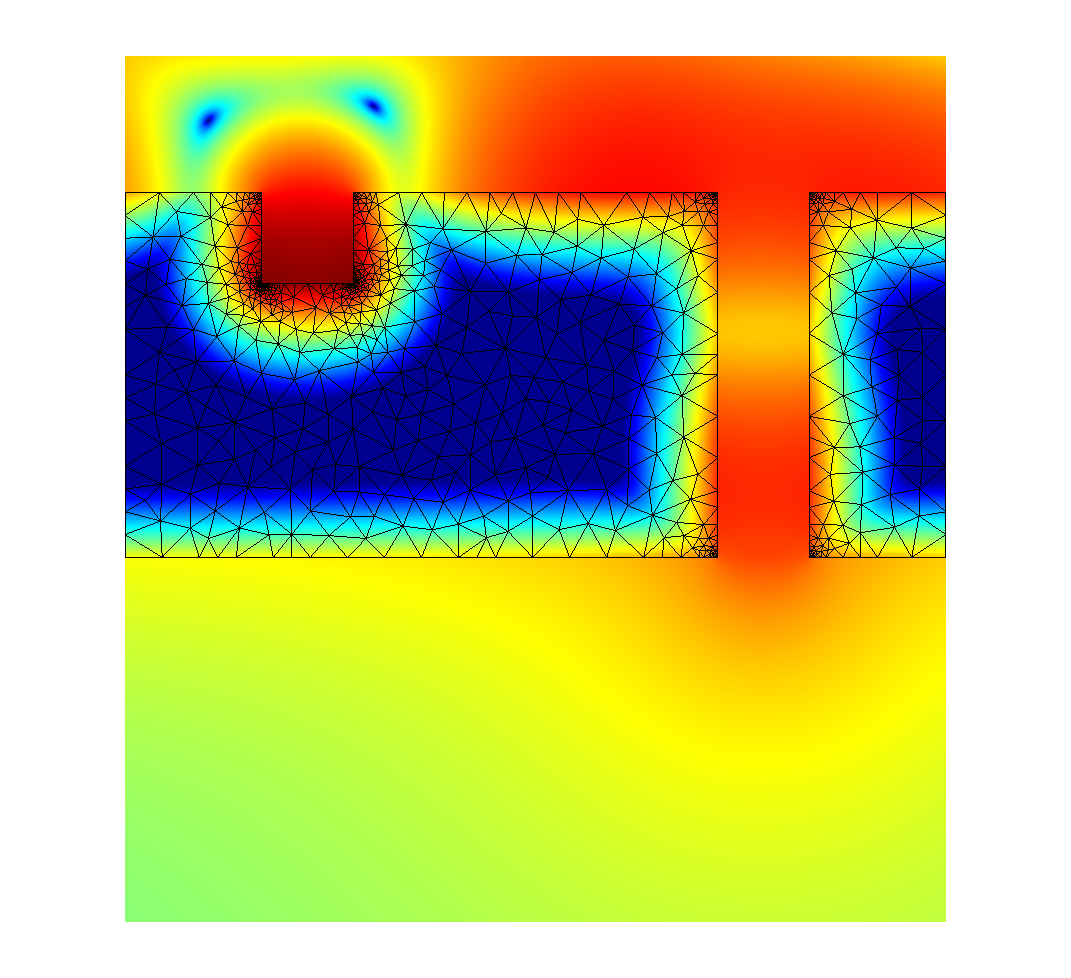# Isolated Slit and Groove¶

This tutorial example follows a benchmark setup investigated by P. Lalanne et al. . Performance of FEM for the same setup has also been demonstrated . The benchmark setup consists of computing the near field in an isolated (i.e., non-periodic) pattern illuminated by a plane wave. The geometry consists of an isolated, sub-wavelength slit in a silver film on a substrate with a neighboring, parallel groove in the silver film. This setup is illuminated by a plane wave at perpendicular incidence from above and with in-plane electric field polarization (resp. out-of-plane magnetic field polarization). The energy flux of light transmitted through the slit to a detector region placed a specific distance below the slit is detected and normalized to the energy flux through the slit, computed in a second simulation where the groove is not present. Due to the geometrical, source and material properties plasmonic effects lead to a very critical dependence of normalized transmission on the physical parameters. This makes accurate computation of normalized transmission a challenging benchmark problem.Definition of the geometry (please note `CornerRefinement` in the definitions for slit and groove):

• layout.jcm [ASCII]

 ``` 1 2 3 4 5 6 7 8 9 10 11 12 13 14 15 16 17 18 19 20 21 22 23 24 25 26 27 28 29 30 31 32 33 34 35 36 37 38 39 40 41 42 43 44 45 46 47 48 49 50 51 52 53 54 55 56 57 58 59 60 61 62 63 64 65 66 67 68 69 70 71 72 73 74 75 76 77 78 79 80 81 82 83 84 85 86 87 88 89 90 91 92 93 94 95 96 97 98 99 100 101 102 103 104 105 106 107 108 109 110 111``` ```Layout2D { UnitOfLength = 1e-09 MeshOptions { MinimumMeshAngle = 28 MaximumSideLength = 50 } Objects { Parallelogram { Name = "CD" DomainId = 1 Priority = ComputationalDomain Width = 900 Height = 950 Boundary { Class = Transparent } } Parallelogram { Name = "Substrate" DomainId = 2 Priority = 3 Width = 900 Height = 400 Port = South Alignment { Parent { Domain = "CD" Port = South } Orientation = Parallel Displacement = [0 0] } } Parallelogram { Name = "Ag" DomainId = 3 Priority = 2 Width = 900 Height = 400 Port = South Alignment { Parent { Domain = "Substrate" Port = North } Orientation = AntiParallel Displacement = [0 0] } } Parallelogram { Name = "Groove" DomainId = 1 Priority = 4 Width = 100 Height = 100 Port = North Alignment { Parent { Domain = "Ag" Port = North } Orientation = Parallel Displacement = [250 0] } MeshOptions { CornerRefinement { MaximumSideLength = 0.1 Progression = 3 } } } Parallelogram { Name = "Slit" DomainId = 1 Priority = 4 Width = 100 Height = 400 Port = North Alignment { Parent { Domain = "Ag" Port = North } Orientation = Parallel Displacement = [-250 0] } MeshOptions { CornerRefinement { MaximumSideLength = 0.1 Progression = 3 } } } Parallelogram { Name = "Detector" DomainId = 4 Priority = 4 Width = 200 Height = 20 Port = South Alignment { Parent { Domain = "Slit" Port = South } Orientation = Parallel Displacement = [0 -400] } } } } ```

Definition of material properties:

• materials.jcm [ASCII]

 ``` 1 2 3 4 5 6 7 8 9 10 11 12 13 14 15 16 17 18 19 20 21 22 23 24 25 26``` ``` Material { Name = "Air" DomainId = 1 RelPermittivity = 1 RelPermeability = 1.0 } Material { Name = "Glass" DomainId = 2 RelPermittivity = 2.25 RelPermeability = 1.0 } Material { Name = "Ag" DomainId = 3 RelPermittivity = (-33.22, 1.17) RelPermeability = 1.0 } Material { Name = "Detector_Glass" DomainId = 4 RelPermittivity = 2.25 RelPermeability = 1.0 } ```

Definition of source properties:

• sources.jcm [ASCII]

 ``` 1 2 3 4 5 6 7 8 9 10 11 12 13 14``` ```SourceBag { Source { MagneticFieldStrength { PlaneWave { Incidence = FromAbove 3DTo2D = yes ThetaPhi = [0 0] Lambda0 = 8.52e-07 SP = [1 0] } } } } ```

Alternatively, the source could be defined as plane wave with `ElectricFieldStrength` and `P` polarization.

Project type, accuracy settings and post-process definitions:

• project.jcmp [ASCII]

 ``` 1 2 3 4 5 6 7 8 9 10 11 12 13 14 15 16 17 18 19 20 21 22 23 24 25 26 27 28 29``` ```Project { InfoLevel = -1 StorageFormat = Binary Electromagnetics { TimeHarmonic { Scattering { FieldComponents = Magnetic Accuracy { FiniteElementDegree = 3 Precision = 0.001 Refinement { MaxNumberSteps = 0 } } } } } } PostProcess { FluxIntegration { FieldBagPath = "project_results/fieldbag.jcm" OutputFileName = "project_results/flux4.jcm" OutputQuantity = ElectromagneticFieldEnergyFlux InterfaceType = ExteriorDomain DomainIdPairs = [4 4] } } ```

The post-process computes the energy flux from the domain with `DomainId = 4` to the adjacent exterior domain with `DomainId = 4`.

The `data_analysis` folder also contains a script for performing simulations with and without the groove (for normalizing the energy flux, according to the benchmark problem). The script also allows to change numerical and physical project parameters, e.g., for checking the accuracy. Some exemplary field distributions are displayed below.

  P. Lalanne, et al., Numerical analysis of a slit-groove diffraction problem, JEOS - Rapid 2, 07022 (2007).
  S. Burger, et al., Finite-element based electromagnetic field simulations: Benchmark results for isolated structures, Proc. SPIE 8880, 88801Z (2013), http://arxiv.org/pdf/1310.2732